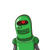# determine wheter the values given against the quadratic equation are the root of the equation or not X2+4x-5=0 ,X=1,-1​

determine wheter the values given against the quadratic equation are the root of the equation or not X2+4x-5=0 ,X=1,-1​

### 1 thought on “determine wheter the values given against the quadratic equation are the root of the equation or not X2+4x-5=0 ,X=1,-1​”

1.